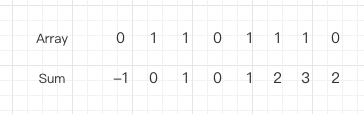# 算法题 leecode 525.连续数组

版权声明 本站原创文章 由 萌叔 发表



### 题目

实例1:


实例2



### 解题思路

• 数组中只会有0、1 两种数据
• 相同数量的 0 和 1 的最长连续子数组中肯定包含有相同数量的0和1

[0, 1, 1, 0, 1, 1, 1, 0]### 最终代码

find_max.go

func findMaxLength(nums []int) int {
// sum -> index
// 存最小值
cache := map[int]int{}
cache = -1
sum := 0
res := 0
for i := 0; i < len(nums); i++ {
if nums[i] == 0 {
sum -= 1
} else {
sum += 1
}
if _, ok := cache[sum]; ok {
index := cache[sum]
res = max(i-index, res)
} else {
cache[sum] = i
}
}
return res
}

func max(a int, b int) int {
if a < b {
return b
} else {
return a
}
}


#### 如果我的文章对你有帮助，你可以给我打赏以促使我拿出更多的时间和精力来分享我的经验和思考总结。